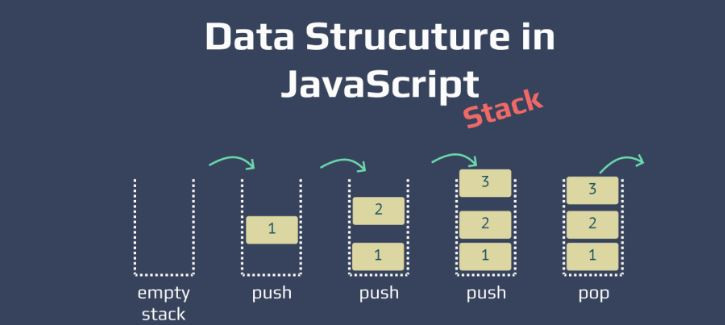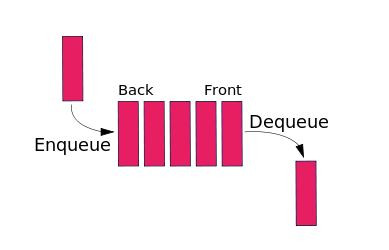DAY 20
0

# 今日kata

Write a function that takes a string of braces, and determines if the order of the braces is valid. It should return true if the string is valid, and false if it's invalid.
This Kata is similar to the Valid Parentheses Kata, but introduces new characters: brackets [], and curly braces {}.
All input strings will be nonempty, and will only consist of parentheses, brackets and curly braces: ()[]{}.

``````"(){}[]"   =>  True
"([{}])"   =>  True
"(}"       =>  False
"[(])"     =>  False
"[({})](]" =>  False
``````

## 構想&解法

``````function validBraces(braces) {
let stack = []
let open = '[({'
let close = '])}'
for (let i = 0; i < braces.length; i++) {
if (open.includes(braces[i])) {
stack.push(braces[i])
} else {
let index = close.indexOf(braces[i]);
if (stack.pop() !== open[index]) return false
}
}
return stack.length === 0 ? true : false
}
``````

## 其他寫法觀摩

``````function validBraces(braces){
while(/\(\)|\[\]|\{\}/g.test(braces)){braces = braces.replace(/\(\)|\[\]|\{\}/g,"")}
return !braces.length;
}
``````

``````function validBraces(braces){
var matches = { '(':')', '{':'}', '[':']' };
var stack = [];
var currentChar;

for (var i=0; i<braces.length; i++) {
currentChar = braces[i];

if (matches[currentChar]) { // opening braces
stack.push(currentChar);
} else { // closing braces
if (currentChar !== matches[stack.pop()]) {
return false;
}
}
}

return stack.length === 0; // any unclosed braces left?
}
``````

## 整理

### 資料結構-Data Structure

• 電話簿
• 圖書館藏書

1. 由先後順序存放資料，想像有張表格，欄位分別是姓名和電話，電話資料由上至下一列一列增加。在這種存放的方式，如果要查詢特定人士的電話，只能逐筆查詢。

2. 由姓氏順序存放資料，想像將`姓氏`當做資料表的索引，在查詢時可由姓氏進行過濾，縮短逐筆查詢的時間。

### Stack(堆疊)及 Queue佇列

Stack為先進後出的特性，生活中像是一疊桌上的盤子，先放的盤子在最底下，最後放入的盤子在最上層，要移除盤子一定要從最上層開始。Queue為先進先出的特性，生活中像是排隊等待上車的乘客，先排隊的人可以先上車。